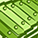# App Development

Announcements
cancel
Showing results for
Did you mean:Contributor III

## Set expression with variable syntax question or a small issue

Hi,

I have inherited one app with a expression syntax that I do not know how to handle.

It is a WTD calculation and works fine this is the expression :

Sum( \$(v_Filter_WTD) Sales )/1000000

This is the expression in the variable

 {\$=' & '\$(v_StartOfWeek)' & '<=' & '\$(v_EndOfWeek)')"},Year=,Month=,Week=,Day=,fYear=>}

So in the calculation I need to add an filter, so I want to add for example StoreNo = 55 into the expression.

I have not managed todo so.

Any hints?

Message was edited by: Birkir Bjornsson

1 Solution

Accepted SolutionsMVP

vTest needs to be without storeNo field in it

\$<DateKey = {"\$(='>=' & '\$(v_StartOfWeek)' & '<=' & '\$(v_EndOfWeek)')"}, Year=, Month=, Week=, Day=, fYear=>

15 RepliesMaster II

Try this:

{\$<DateKey={"\$(='>=' & '\$(v_StartOfWeek)' & '<=' & '\$(v_EndOfWeek)')"},StoreNo={'55'},Year=,Month=,Week=,Day=,fYear=>}

HTH

SushilContributor III
Author

Hi Sushil,

Thanks for your valid input, yes this solves this. But I was hoping to be able to have the WTD filter universal and add the StoreNo = 55

into this expression

Sum( \$(v_Filter_WTD) Sales )/1000000, that I have not been able to solve.

Any ideas?Champion III

Vineeth Pujari
If a post helps to resolve your issue, please accept it as a Solution.MVP

May be this:

Sum({\$<DateKey={"\$(='>=' & '\$(v_StartOfWeek)' & '<=' & '\$(v_EndOfWeek)')"},StoreNo={'55'},Year=,Month=,Week=,Day=,fYear=>}

Aggr(

Sum( \$(v_Filter_WTD) Sales )/1000000

, YourChartDimensionsHere)Contributor III
Author

Hi all,MVP

What is the expected output here?Contributor III
Author

Well the idea here would bet do add an storeno filter into the expression in the top KPI,

So for example set StoreNo = 24 should show 15.08K in the Kpi as WTD sales.

So is it somehow possible to add storeno into the set analysis using the wtd variable as is, So that could be an universal variable and then add whatever extra filters you need into the set analysis.

does that makes sense?MVP

May be like this?

Sum({\$<DateKey = {"\$(='>=' & '\$(v_StartOfWeek)' & '<=' & '\$(v_EndOfWeek)')"}, StoreNo ={'24'}, Year=, Month=, Week=, Day=, fYear=>} Aggr(Sum( \$(v_Filter_WTD) Sales )/1000000, DateKey))

or if you can define your variables like this (without the curly brackets at the start and end)

\$<DateKey = {"\$(='>=' & '\$(v_StartOfWeek)' & '<=' & '\$(v_EndOfWeek)')"}, StoreNo ={'24'}, Year=, Month=, Week=, Day=, fYear=>

then this might work

Sum({\$(v_Filter_WTD)*<StoreNo ={'24'}>} Sales)/1000000MVP

Tested the second one with a new variable called vTest